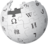# Bessel function

From Halopedia, the Halo wikiThere is more information available on this subject at Bessel function on the English Wikipedia.

Bessel functions, in mathematics, first defined by the mathematician Daniel Bernoulli and generalized by Friedrich Bessel, are canonical solutions y(x) of Bessel's differential equation:

x^2 \frac{d^2 y}{dx^2} + x \frac{dy}{dx} + (x^2 - \alpha^2)y = 0

For an arbitrary real or complex number α. The most common and important special case is where α is an integer n, then α is referred to as the order of the Bessel function.

Although α and −α produce the same differential equation, it is conventional to define different Bessel functions for these two orders (e.g., so that the Bessel functions are mostly smooth functions of α). Bessel functions are also known as Cylinder functions or Cylindrical harmonics because they are found in the solution to Laplace's equation in cylindrical coordinates.

## Usage

The artificial intelligence aboard the Ascendant Justice attempted to use Bessel functions to communicate with Cortana. Dominique had a tattoo of a Bessel function on his left wrist.